# Introduction

We are deprecating `residPlot()` from the next version of FSA (v0.9.0). It will likely be removed at the end of the year 2001. We are taking this action to make `FSA` more focused on fisheries applications and to eliminate “black box” functions. `residPlot()` was originally designed for students to quickly visualize residuals from one- and two-way ANOVAs and simple, indicator variable, and logistic regressions.1

We now feel that students are better served by learning how to create these visualizations using methods provided by `ggplot2`, which require more code, but are more modern, flexible, and transparent.

The basic plots produced by `residPlot()` are recreated here using `ggplot2` to provide a resource to help users that relied on `residPlot()` transition to `ggplot2`.

The examples below require the following additional packages.

Most examples below use the `Mirex` data set from `FSA`, which contains the concentration of mirex in the tissue and the body weight of two species of salmon (`chinook` and `coho`) captured in six years. The `year` variable is converted to a factor below for modeling purposes. These same data were used in this post about depredating `fitPlot()`.

# One-Way ANOVA

The code below fits a one-way ANOVA model to examine if mean weight differs by species.

`residPlot()` from `FSA` (before v0.9.0) produces a boxplot of residuals by group (left) and a histogram of residuals (right).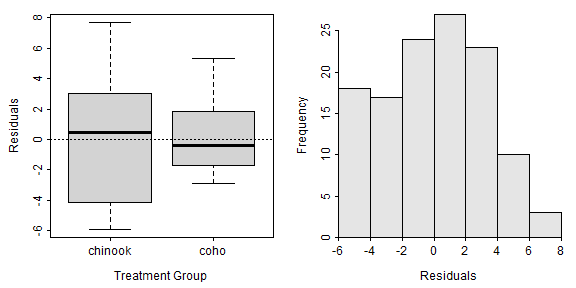A data.frame of the two variables used in the ANOVA appended with the fitted values and residuals from the model fit must be made to construct this plot using `ggplot()`. Studentized residuals are included below in case you would prefer to plot them.2

The histogram of residuals is constructed with `geom_histogram()` below. Note that the color of the histogram bars are modified and the bin width is set to better control the number of bars in the histogram. Finally, the bottom multiplier for the y-axis is set to zero so that that histogram bars do not “hover” above the x-axis.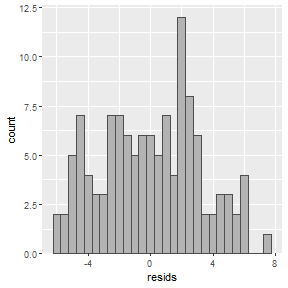The boxplot of residuals by group (species in this case) is constructed with `geom_boxplot()` below (again controlling the colors of the boxplot).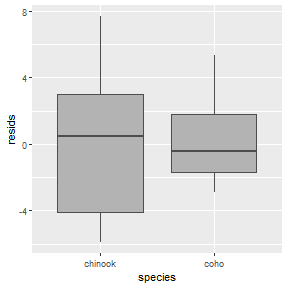These plots can be further modified using methods typical for ggplot (see conclusion section).

# Two-Way ANOVA

The code below fits a two-way ANOVA model to examine if mean weight differs by species, by year, or by the interaction between species and year.

`residPlot()` from `FSA` (before v0.9.0) shows a boxplot of residuals by each combination of the two factor variables (left) and a histogram of the residuals (right).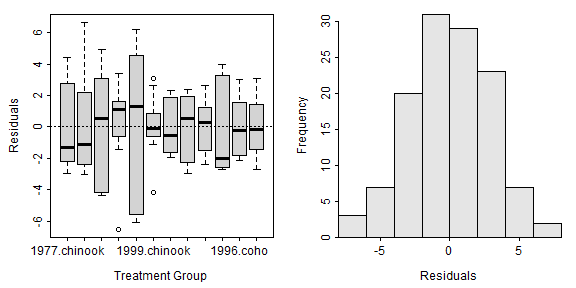A data.frame of the three variables used in the ANOVA appended with the fitted values and residuals from the model fit must be constructed.

The histogram of residuals is constructed exactly as before and won’t be repeated here. The boxplot of residuals by group is constructed with one of the factor variables on the x-axis3 and the other factor variable as separate facets.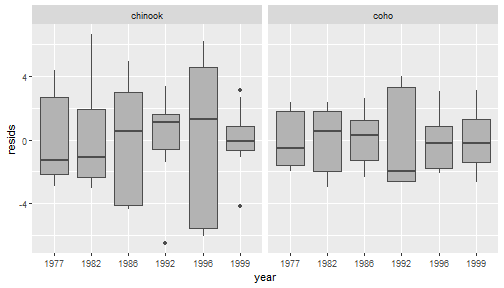# Simple Linear Regression

The code below fits a simple linear regression for examining the relationship between mirex concentration and salmon weight.

`residPlot()` from `FSA` (before v0.9.0) shows a scatterplot of residuals versus fitted values (left) and a histogram of residuals (right).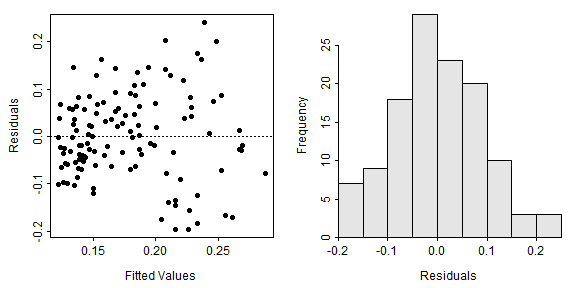A data.frame of the two variables used in the ANOVA appended with the fitted values and residuals from the model fit must be constructed.

The histogram of residuals is constructed exactly as before and won’t be repeated here. The scatterplot of residuals versus fitted values is constructed with `geom_point()` as below. Note that `geom_hline()` is used to place the horizontal line at 0 on the y-axis.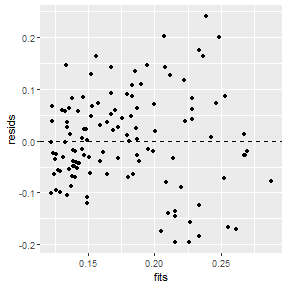It is also possible to include a loess smoother to help identify a possible nonlinearity in this residual plot.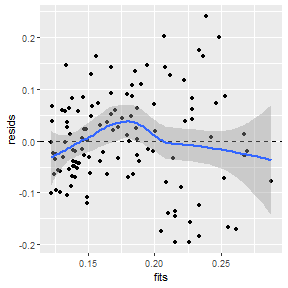## Indicator Variable Regression

The code below fits an indicator variable regression to examine if the relationship between mirex concentration and salmon weight differs betwen species.

`residPlot()` from `FSA` (before v0.9.0) is the same for an IVR as for an SLR, except that the points on the residual plot (left) has different colors for the different groups.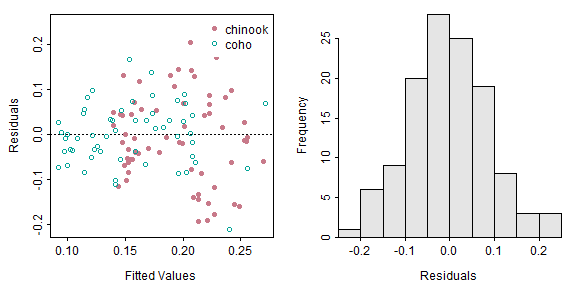A data.frame of the three variables used in the ANOVA appended with the fitted values and residuals from the model fit must be constructed.

The histogram of residuals is constructed exactly as before and won’t be repeated here. The scatterplot of residuals versus fitted values is constructed with `geom_point()`. Note that `color=` and `shape=` are both set equal to the factor variable to change the color and plotting character to represent the different groups.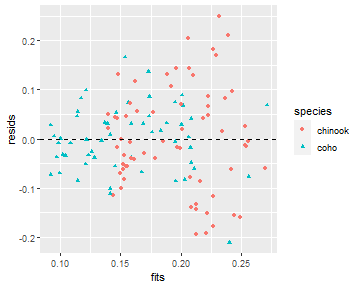## Nonlinear Regression

The following code fits a von Bertalanffy growth function (VBGF) to the total length and age data for spot found in the `SpotVA1` data frame built into `FSA`. Fitting the VBGF is described in more detail here.

`residPlot()` from `FSA` (before v0.9.0) produces plots excatly as for a simple linear regression.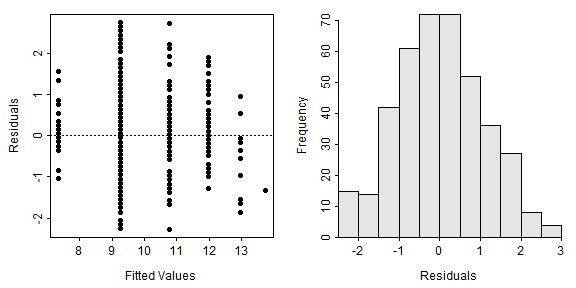A data.frame of the two variables used in the ANOVA appended with the fitted values and residuals from the model fit must be constructed. The `rstudent()` function does not work for non-linear models, but the Studentized residuals are computed with `nlsResiduals()` from `nlstools`. However, these values are “buried” in the `Standardized residuals` column of the `resi2` matrix returned by that function.

Once this data frame is contstructed the residual plot and histogram of residuals are constructed exactly as before and won’t be repeated here.

## Conclusion

The `residPlot()` function in `FSA` will be deprecated in v0.9.0 and will likely not exist after that. This post describes a more transparent (i.e., not a “black box”) and flexible set of methods for constructing similar plots using `ggplot2` for those who will need to transition away from using `residPlot()`. It should also be noted that different “residual plot” functionality is available in `plot()` (from base R when given an object from `lm()`), `car::residualPlots()`, `DHARMa::plotResiduals()`, and `ggResidpanel::resid_panel()`.

As mentioned in the examples above, each plot can be modified further using typical methods for `ggplot2`. These changes were not illustrated above to minimize the amount of code shown in this post. However, as an example, the code below shows a possible modification of the IVR residual plot shown above. Note that the `patchwork` package is needed to place the plots side-by-side.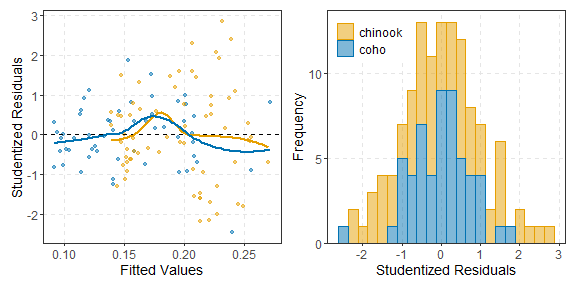## Footnotes

1. Over time functionality for non-linear regressions was added.

2. These are “internally” Studentized residuals. “Externally” Studentized residuals can be obtained with `rstandard()`

3. These two variables can, of course, be exchanged. However, I generally prefer to have the variable with more levels on the x-axis.